Also found in: Dictionary, Thesaurus, Medical.

Classical radiation theory (Maxwell’s theory). The physical reasons for the existence of a free electromagnetic field (that is, a self-sustaining field that has become independent of the sources that induced it) are closely associated with the fact that electromagnetic waves propagate from sources—charges and currents —with a finite speed c (in a vacuum c˜3 X 1010 cm/sec) rather than instantaneously. If the radiation source (such as an alternating current) disappears at a certain instant, the field will not disappear instantaneously in the entire space: at points remote from the source it will disappear only after a finite time interval. It follows from Maxwell’s theory that a change in an electric field E in time gives rise to a magnetic field H, and the change in H gives rise to a vortex electric field. Hence it follows that only a variable electromagnetic field, in which both components—E and H—constantly excite each other by changing continuously, may be self-sustaining.

In the process of radiation an electromagnetic field carries energy away from the source. The energy flux density of the field (the quantity of energy per unit time passing through a unit area oriented perpendicular to the direction of the flux) is determined by the Poynting vector P, which is proportional to the vector product [EH].

The radiation intensity Eradis the energy removed from the source by the field per unit time. Its order of magnitude can be estimated by calculating the product of the area of the closed surface encompassing the source and the average value of the absolute flux density P on this surface (P˜EH). The surface is usually selected in the form of a sphere of radius R (its area is on the order of R2), and Erad is calculated from the limit R → ∞:

(E and H are the absolute values of the vectors E and H).

In order that this quantity does not disappear—that is, in order for a free electromagnetic field to exist far from the source —both E and H must not decrease more rapidly than \/R. This requirement is satisfied if moving charges under acceleration are the field sources. Near the charges the fields are Coulomb fields proportional to l/R2, but at large distances non-Coulomb fields Eand H, which attenuate as l/R, begin to play the primary role.

RADIATION OF A MOVING CHARGE. A point charge is the simplest field source. A resting charge has no radiation; nor may a uniformly moving charge (in a vacuum) be a source of radiation. However, a charge moving under acceleration does radiate. Direct calculations based on Maxwell’s equations show that the intensity of its radiation is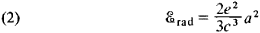where e is the magnitude of the charge and a is its acceleration. (Here and below the Gaussian system of units is used.) Depending on the physical nature of the acceleration the radiation is sometimes given special names. For example, the radiation that arises during the deceleration of charged particles in matter as a result of the action of the Coulomb fields of automic nuclei and electrons is called bremsstrahlung. The radiation of a charged particle moving in a magnetic field that deflects its trajectory is called synchrotron radiation. It is observed, for example, in cyclic charged-particle accelerators.

In a particular case, when a charge undergoes a harmonic oscillation, the acceleration a is equal in magnitude to the product of the deviation of the charge from a state of equilibrium (x = x0 sin ωt, where x0 is the amplitude of the deviation of x) and the square of the frequency s. The radiation intensity, averaged with respect to time t, increases very rapidly (proportional to ω4) as the frequency increases: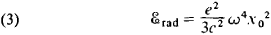ELECTRIC DIPOLE RADIATION. Two interconnected, co-oscillating opposite charges that are equal in magnitude are the simplest system that may serve as a source of radiation. They form a dipole with a variable moment. For example, if the charges of the dipole oscillate harmonically against each other, then the electric dipole moment changes according to the law d = d0 sin wt (ω is the frequency of oscillations, and do is the amplitude of moment d). The radiation intensity of such a dipole averaged with respect to the time t is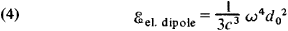The radiation emanating from an oscillating dipole is anisotropic—that is, the energy emitted in various directions is not identical. Radiation is entirely absent along the axis of oscillations and is at a maximum at right angles to the axis of oscillations. For all intermediate directions the angular distribution of the radiation changes in proportion to sin2θ, where the angle θ is read from the direction of the axis of oscillations. If the direction of the axis of oscillations of a dipole changes over time, then the average angular distribution becomes more complex.

Real radiators usually include a set of charges. Precise consideration for all details of the motion of each charge in the study of radiation is unnecessary and often impossible. Indeed, radiation is defined by the values of the fields far from the source— that is, where the details of the distribution of the charges and currents in the radiator have a slight effect. This makes it possible to replace the true distribution of the charges with an approximate distribution. Consideration of a radiating system as a single charge, equal in magnitude to the sum of the charges of the system, is the crudest, “zero-order” approximation. In an electrically neutral system, the sum of whose charges is equal to zero, radiation is absent in this approximation. In the next approximation (the first), the positive and negative charges of the system “tend” imaginarily toward the centers of their distribution. For an electrically neutral system this means conceptual replacement by an electric dipole radiating according to (4). This approximation is called a dipole approximation, and the corresponding radiation is called electric dipole radiation.

ELECTRIC QUADRUPOLE AND HIGHER MULTIPOLE RADIATION. If a system of charges has no dipole radiation—for example, because the dipole moment is equal to zero—then it is necessary to take into account the next approximation, in which the system of charges (the radiation source) is regarded as a quadrupole. The simplest quadrupole is two dipoles that have moments of equal magnitude and opposite direction. An even more detailed description of a radiating system of charges is given by consideration of subsequent approximations, in which the charge distribution is described by multipoles of higher orders (a dipole is called a first-order multipole, a quadrupole a second-order multipole, and so on).

It is important to note that in each successive approximation the radiation intensity is approximately (c/v)2 times less than in the previous one (if, of course, it is not absent for some reason). If the radiator is nonrelativistic—that is, if all charges have velocities much less than the speed of light (v/c « 1)—then the lower, nonvanishing approximation plays the main role. Thus, if dipole radiation is present it is the primary radiation, and all other higher multipole corrections are extremely small and need not be taken into account. In the case of the radiation of relativistic particles, however, the description of radiation by means of multipoles becomes ineffective, since the contribution of higher-order multipoles ceases to be small.

MAGNETIC DIPOLE RADIATION. In addition to electric dipoles and higher multipoles, magnetic dipoles and multipoles may also be radiation sources (magnetic dipole radiation is generally the primary radiation). The distribution pattern of a magnetic field at a great distance from the circuit through which the current that gives rise to this field is flowing is similar to the distribution pattern of an electric field far from an electric dipole. The analogue of the electric dipole moment, the dipole magnetic moment M, is determined by the current strength I in the circuit and by the circuit’s geometry. For a plane circuit the absolute magnitude of the moment is M = (e/c )IS, where S is the area within the circuit. The formulas for the intensity of magnetic dipole radiation are almost the same as for electric radiation, except that they contain the magnetic moment M instead of the electric dipole moment d. Thus, if the magnetic moment changes according to the harmonic law M = M0 sin cat (for this the current strength I in the circuit must change harmonically), the radiation intensity averaged with respect to time is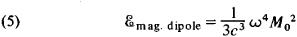Here M0 is the amplitude of the magnetic moment M.

The ratio of the magnetic and electric dipole moments is of the order of v/c, where v is the rate of motion of the charges forming the current; from this it follows that the intensity of magnetic dipole radiation is (c/v)2 times less than that of electric dipole radiation if, of course, the latter is present. Thus, the intensities of magnetic dipole and electric quadrupole radiation are of the same order of magnitude.

RADIATION OF RELATIVISTIC PARTICLES. The synchrotron radiation of charged particles in circular (ring) accelerators is one of the most important examples of radiation of relativistic particles. A sharp difference from nonrelativistic radiation is manifested here in the spectral composition of the radiation: if the revolution frequency of a charged particle in an accelerator is equal to ω (a nonrelativistic radiator would emit waves of the same frequency), then its radiation intensity has a maximum at a frequency ωmax ˜ ³3ω, where ³ = [1 — (v/c)2]-1/2; that is, when vc most of the radiation is found at frequencies higher than ω. Such radiation is directed nearly at a tangent to the orbit of the particle, mainly in the direction of its motion.

An ultrarelativistic particle may radiate electromagnetic waves even if it is moving in a straight line and uniformly (but only in matter, not in a vacuum!). This radiation, called Cherenkov radiation, arises if the velocity of a charged particle in a medium exceeds the phase velocity of light in this medium (uPhase = c/n, where n is the index of refraction of the medium). The radiation appears because the particle “overtakes” and breaks away from the field that gives rise to it.

Quantum radiation theory. It is has already been stated above that classical theory gives only an approximate description of radiation processes (the entire physical world is in principle a “quantum” world). However, physical systems also exist whose radiation cannot be described even approximately in conformance with experiments from the standpoint of classical theory. An important feature of such quantum systems as the atom or molecule is that their internal energy does not change continuously but may assume only certain values, forming a discrete set. The transition of a system from a state with one energy to a state with another takes place in a stepwise manner; because of the law of conservation of energy, upon such a transition a system must lose or acquire a certain “portion” of energy. This process is realized most often in the form of emission or absorption of a quantum of radiation—a photon—by the system. The energy of a quantum is Є³ =ħω where ħ is Planck’s constant (ħ = 1.05450 X 10-27 erg/sec), and ω is the angular frequency. A photon always acts as a unified whole, is emitted and absorbed “in its entirety,” in a single event, and has a definite energy, momentum, and spin (the projection of the angular momentum on the direction of the momentum)—that is, it has a number of corpuscular properties. At the same time the photon differs sharply from conventional classical particles in that it also has wave features. This dual character of the photon is a particular phenomenon of particle-wave dualism.

Quantum electrodynamics is a consistent quantum theory of radiation. However, many results that bear on the processes of the radiation of quantum systems can be obtained from the simpler semiclassical radiation theory. The formulas of the latter, according to the correspondence principle, should, within certain limits, give the results of classical theory for some limiting transition. Thus, a profound analogy is established between the quantities that characterize radiation processes in quantum and classical theories.

ATOMIC RADIATION. A system consisting of a nucleus and an electron moving in its Coulomb field must be in one of the discrete states (at a certain energy level). All states except the ground state (the state with the lowest energy) are unstable. An atom in an unstable (excited) state, even if isolated, passes into a state of lower energy. This quantum transition is accompanied by the emission of a photon; such radiation is called spontaneous radiation. The energy removed by the photon, Єϒ = ħω, is equal to the difference between the energies of the initial state and the final state j of the atom (ЄiЄj; Єi—Єj); the formula of N. Bohr for radiation frequencies follows from this: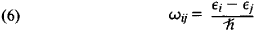It is important to note that such characteristics of spontaneous radiation as the direction of propagation (for a set of atoms, the angular distribution of their spontaneous radiation) and polarization do not depend on the radiation of other objects, such as an external electromagnetic field.

Bohr’s formula (6) defines the discrete set of frequencies, and consequently the wavelengths, of atomic radiation. It explains why the spectra of atomic radiation have the well-known “line” character—each line of the spectrum corresponds to one of the quantum transitions of the atoms of the particular substance.

RADIATION INTENSITY. In quantum theory, as in classical theory, electric dipole and higher multipole radiation may be examined. If a radiator is nonrelativistic, the primary radiation is the electric dipole radiation whose intensity is determined by a formula close to the classical formula: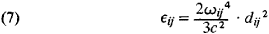The quantities dij, which are a quantum analogue of the electric dipole moment, are found to be nonzero only when certain relations exist between the quantum numbers of the initial state /’ and the final state j (the selection rules for dipole radiation). Quantum transitions that satisfy such selection rules are called allowed transitions (the allowed electric dipole radiation is actually meant). By contrast, transitions of higher multipole levels are said to be forbidden. This prohibition is relative: forbidden transitions have a relatively low probability—that is, the radiation intensity corresponding to them is small. The states from which transitions are “forbidden” are comparatively stable (long-lived); they are called metastable states.

Quantum radiation theory makes possible an explanation not only of the difference in intensity of various lines but also of the intensity distribution within each line, and in particular the width of spectral lines.

Not only atoms but also more complex quantum systems may be sources of electromagnetic radiation. The general methods for describing the radiation of such systems are the same as those used in the study of atoms, but the specific features of the radiation are extremely diverse. For example, molecular radiation has more complex spectra than does atomic radiation. High energy of individual quanta (³-quanta) and comparatively low radiation intensity are typical of the radiation of atomic nuclei.

Electromagnetic radiation frequently also arises during mutual transformations of elementary particles (such as the annihilation of electrons and positrons and the decay of the neutral pi-meson).